• 3 + 2 = 5 is an example of an addition number sentence.
• The + sign is called a plus sign or an addition sign.
• We combine the numbers on either side of the plus sign together to make a total.
• In the example of 3 + 2, the plus sign means to combine the 3 and the 2 together.
• In total, 3 counters plus 2 more counters make 5 counters.
• The = sign is called the equals sign.
• In a number sentence, the equals sign means that the total value on the left of the equals sign is the same as the total value on the right of the equals sign.
• It means that having 3 counters and then 2 more is the same as simply having 5 counters.
‘+’ is called the plus sign and it means to combine the values of the numbers that are either side of it.

‘=’ is called the equals sign and it means that the total of the numbers on either side of it are the same in value.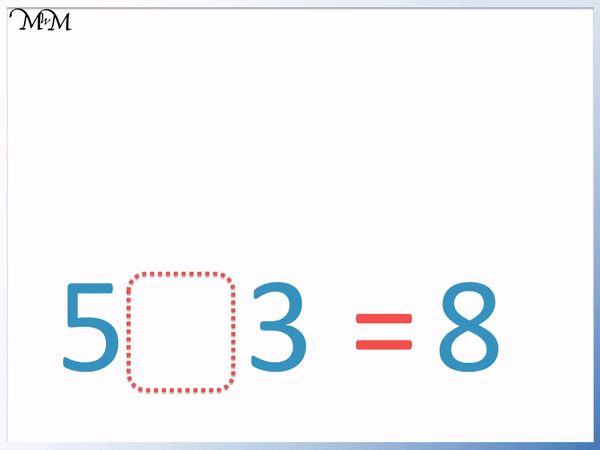• In this addition number sentence example we have the values of 5, 3 and 8.
• Each number in a number sentence must be separated by a mathematical symbol.
• We can see that 3 and 5 counters combined together on the left is the same value as the 8 counters on the right.
• The equals sign tells us that this is the case.
• The mathematical symbol that tells us to combine the two numbers together into a total is the plus sign.
• The plus sign or addition sign is ‘+’ and this must go in between the two numbers that we wish to add together.
• Our number sentence is 5 + 3 = 8 and is read as ‘five add three equals eight’.# How do we Write Addition Number Sentences?

A number sentence is just a combination of numbers and mathematical symbols.

Some common mathematical symbols used in number sentences are:

• The plus sign (or addition sign): ‘+’
• The minus sign (or subtraction sign): ‘-‘
• The equals sign: ‘=’
• The less-than sign: ‘<'
• The greater-than sign: ‘>’

In this lesson we are only looking at addition number sentences, which will only contain the plus sign ‘+’ and the equals sign ‘=’.

Below is our first example of forming an addition number sentence.We also have two more counters.

We can collect all of the counters together and count them to see how many we have altogether in total.

Counting the counters, we have 5 in total. Three counters and two more makes a total of 5 counters.

We say that we have added three and two together.

Instead of writing down ‘three add two’ it is quicker to write ‘3 + 2’, where the ‘+’ sign is called a plus sign or an addition sign.

When teaching addition to children it is important to remember that the plus sign must separate the two numbers that we wish to combine together.The next sign that we use is an equals sign, which is written as ‘=’.

Here the equals sign tells us that 3 and 2 combined together is the same as 5. Writing an equals sign means that the total value on the left of the equals sign is the same as the total value on the right of the equals sign.

It tells us that if we are given 3 counters and then 2 more counters, it is exactly the same as being given 5 counters straight away.

We use an equals sign to show that we have a correct number sentence.

We can see below that it is ok to write and equals sign between 3 + 2 and 5 but not in between 3 + 2 and 9.

We would actually use the ‘not equal to’ sign: ‘≠’ to show that 3 + 2 does not equal 9.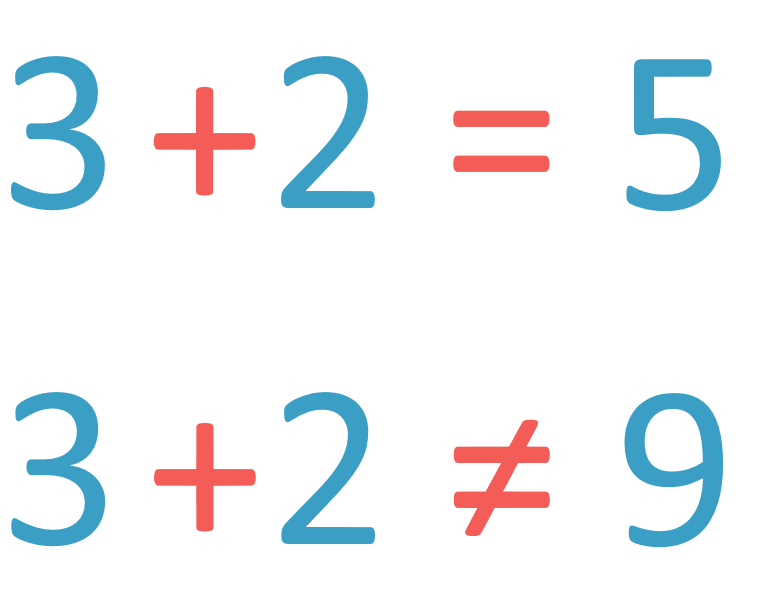Below is another example of a correct addition number sentence.

We have 4 counters and then 3 more.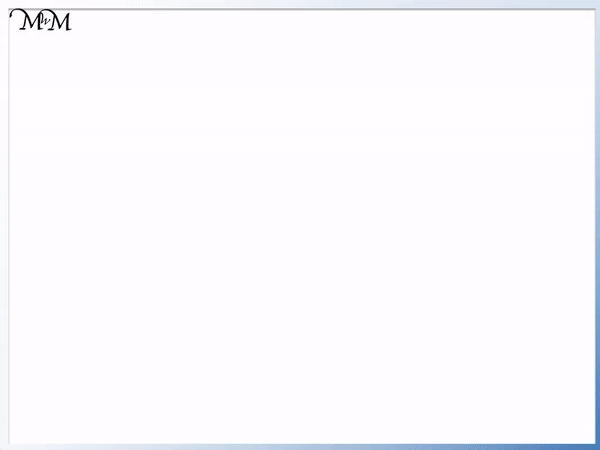We will add the 4 counters to the three counters. Remember that this means that we want to see how many counters that we have in total and we write a plus sign ‘+’ in between the 4 and the 3.

If we count the total number of counters, we can see that there are 7.

To show that 4 add 3 is the same value as 7, we write an equals sign in between the 4 + 3 and the 7.

It is very common that the equals sign will appear directly before the ‘answer’ of a sum and whilst it can be helpful to think of the equals sign as meaning ‘the answer is’, it just means that whatever is on the left of this sign is the same value as whatever is on the right of the equals sign.

For example we could have written the addition number sentence the other way around: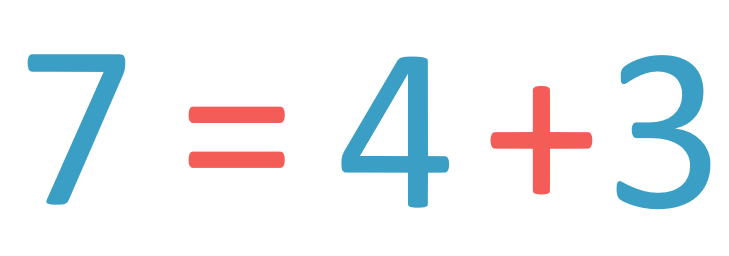Whilst it is not so common in school questions to have one number on the left of an equals sign and more than one number on the right, this is still a completely correct addition number sentence.

It just states that seven is the same value as four plus three more.

Furthermore, it is possible to have an addition on both sides of the equals sign, such as in the example below: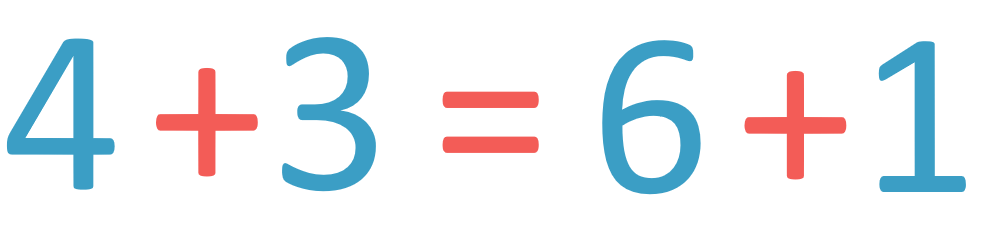4 + 3 = 6 + 1 means that having 4 counters and then being given more 3 counters is exactly the same as having 6 counters and being given one more.

Either way, we have 7 counters. Both sides of the equals sign are equal to 7 and so an equals sign can be used to separate the left and right sides.

In the example below we have all of the numbers in our addition number sentence.

We have three numbers given to us, 2 , 2 and 4.

We are asked to completed the number sentence by filling in the blank.

It cannot be another number that goes in this box.

We know this since we do not put two numbers directly next to each other in a number sentence. We separate numbers with mathematical symbols.We can see that we have 2 + 2. The plus sign tells us that we are combining the two numbers directly either side of the + sign together to make a total.

2 + 2 is 4 in total.

We have a 4 written in the number sentence at the end on the right. 2 + 2 is the same value as 4 and so we show this by writing an equals sign in between 2 + 2 and 4.

In this last example we are given three numbers: 5, 3 and 8.

We are asked to complete the number sentence by filling in the box in between the 5 and the 3.

Remember that we cannot put another number here directly next to other numbers. Therefore we are choosing a mathematical symbol to go here.We can see that combining 5 counters with 3 counters is exactly the same as having 8 counters.

The word for the process of combining these counters to make a total is addition.

We add the 5 and the 3.

And so we put an addition sign or a plus sign in between the 5 and the 3 to show that we are combining them to make a total that is equal to 8.

This addition number sentence is read as ‘five add three equals eight’ and it means that combining 5 counters with 3 more counters is the same as simply having 8 counters from the start.Now try our lesson on Subtraction Number Sentences where we learn how to write number sentences for subtraction.